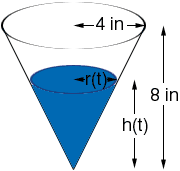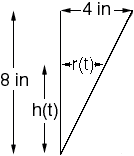Hello my name is Sarah. I am a secondary student, actually a 12th grader in high school. I am having a hard time figuring out the formulas for the following problem from my claculus I class. The problem: Water flows into a conical funnel at a continuous rate of one gallon per minute (One gallon = 231 Cu.In.). The height of the funnel is 5" and the diameter is 8". The 1st formula: I need to develop a formula that will give the volume, in cubic inches, of the water in the funnel at any time t (in seconds). V = f(t). The 2nd formula: I need to develop a formula that will give the height of the water in the funnel at any time t (in seconds). h = f(t). Thank you so much for helping me. Sarah Hi Sarah, The fact you need is an expression for the volume of a cone if you know its height and the radius of the top. The expression is V = 1/3 pi r2 h where V is the volume of the cone, h is its height and r is the radius of the top. (This is one-third the volume of the cylinder with the same radius and base.) A diagram of the funnel with some water in it is below.I have called the height of the water h(t) and the radius of the top of the water r(t). These are both functions of t and change as water flows into the funnel. If you imagine a line from the center of the circle at the top of the cone to the vertex of the cone you form two triangles.These triangles are similar so  h(t)/8 = r(t)/4 Can you finish the problem now? Penny Go to Math Central